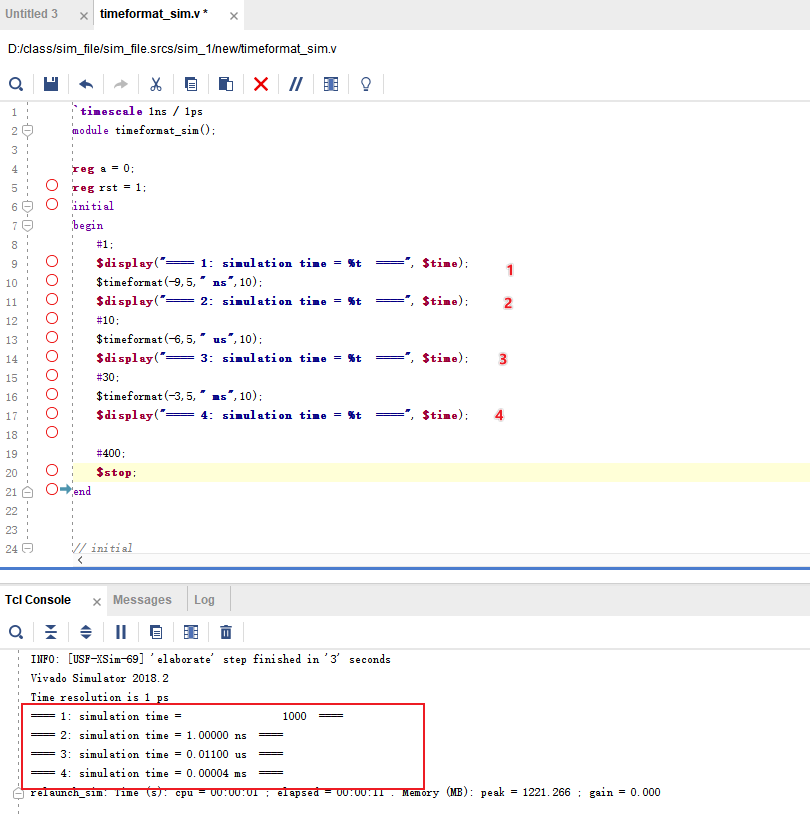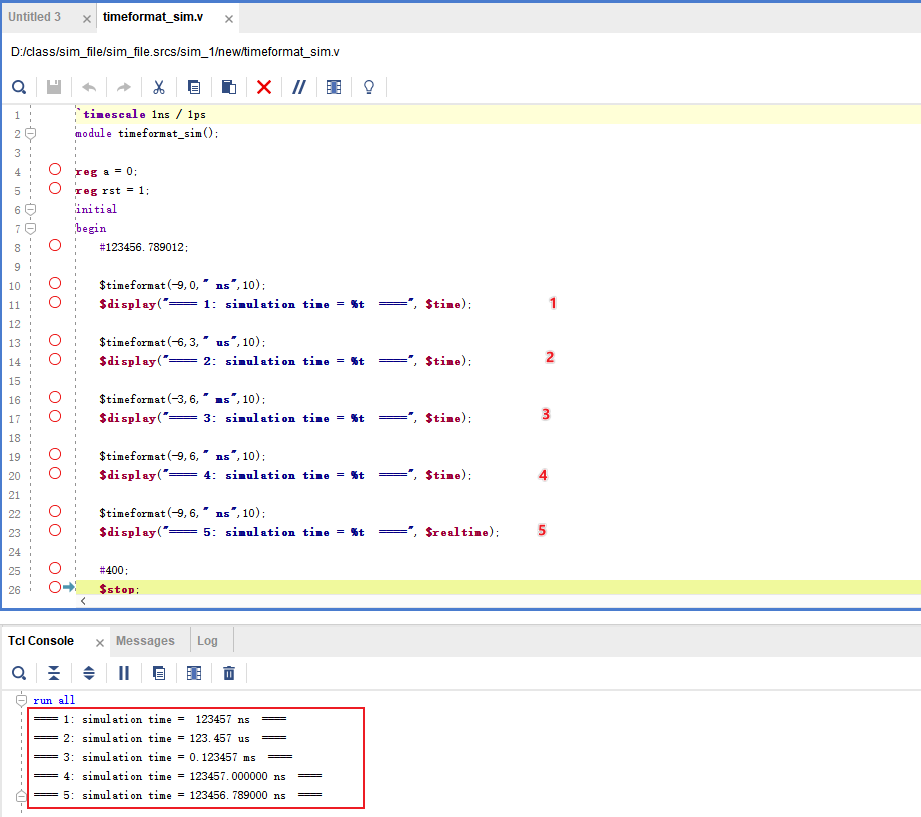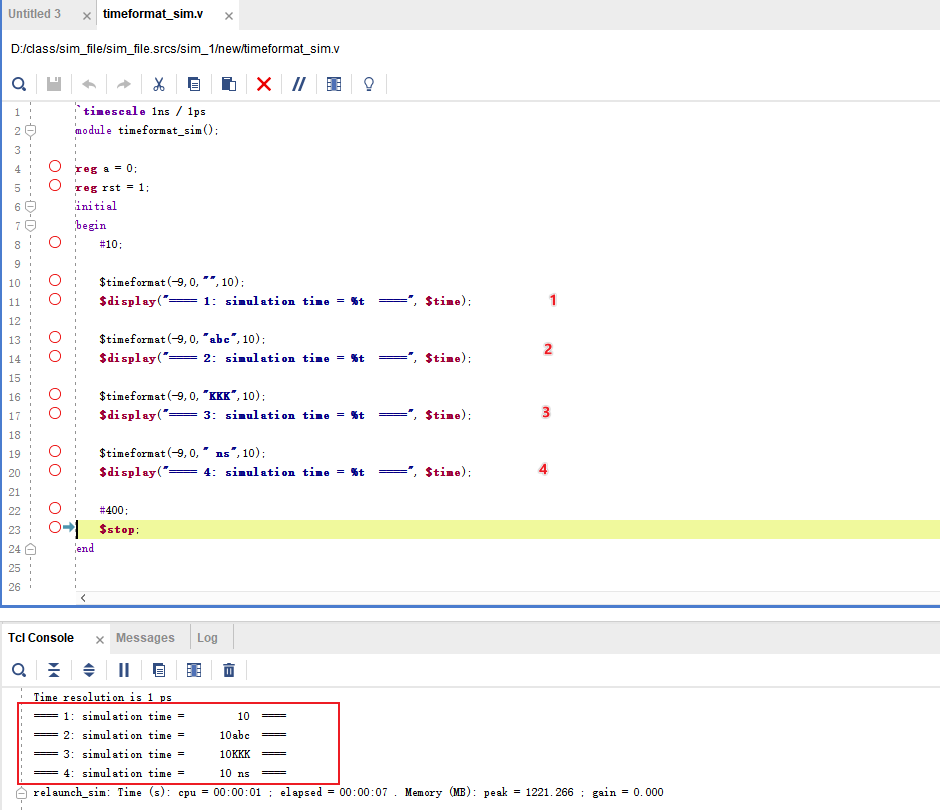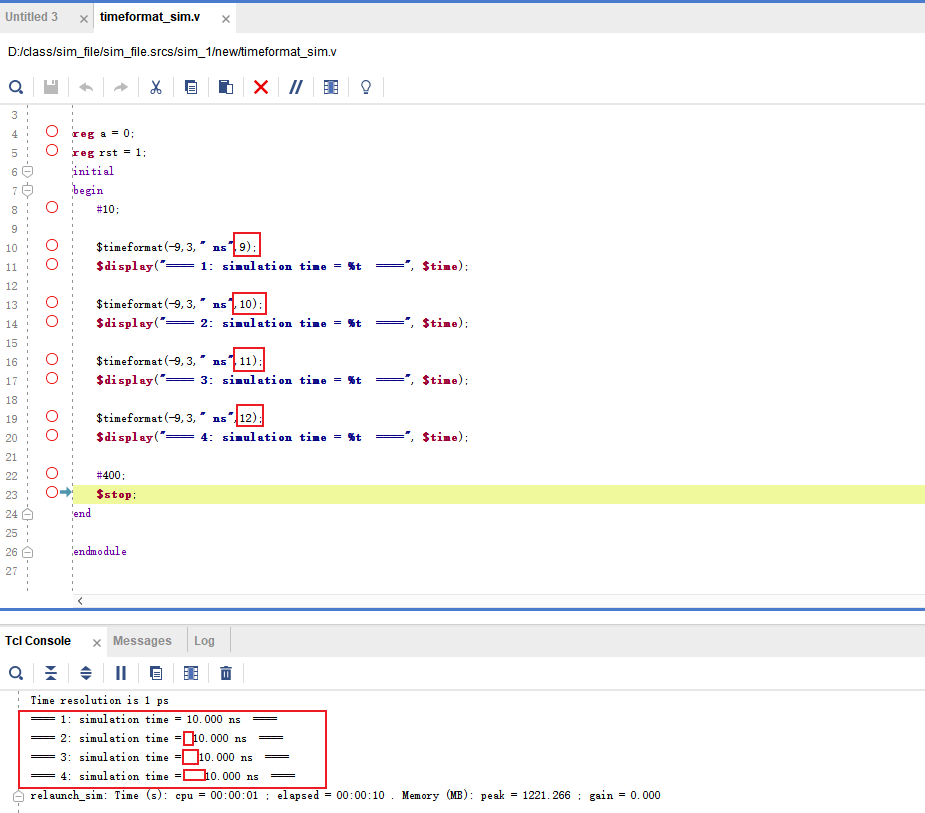# Verilog 仿真中 \$timeformat 使用

````timescale 1ns / 1ps

module tb(
);

reg a = 0;
reg rst = 1;
initial
begin
#200;
rst = 1;
#10;
rst = 0;
\$display("==== init a = 0, time = %t  ====", \$time);
end

endmodule
```

### 1 \$timeformat 任务格式

`\$timeformat ( units_number, precision_number, suffix_string, minimum_field_width) ;`

• units_number: 是 0 到-15 之间的整数值，表示格式化输出的时间值的单位：0 表示秒，-3 表示毫秒，-6 表示微秒，-9 表示纳秒；它是以10（units_number）幂秒表示当前输出的时间单位。例如 -3 ：代表%t 输出是以ms 为时间单位输出的，即 10-3 s = 1ms。具体数字所代表的含义，见表1

 Unit number Time unit Unit number Time unit 0 1 s -8 10 ns -1 100 ms -9 1 ns -2 10 ms -10 100 ps -3 1 ms -11 10 ps -4 100 us -12 1 ps -5 10 us -13 100 fs -6 1 us -14 10 fs -7 100 ns -15 1 fs
• precision_number: 是指打印输出时， 小数点后保留多少位10进制数
• suffix_string: 后缀字符串， 可以增加 打印输出的时间单位。 例如 ” ps”   ” ns”  ” us” ” ms” 等。这样就不需要在 向 \$display 等函数中额外添加时间单位了。
• minimum_field_width: 是指打印输出的时间值字符串+suffix_string 字符串的最小长度，如果时间值字符串+suffix_string 字符串 小于 minimum_field_width，则在时间值字符串+suffix_string字符串之前补空格。注：这个功能只对%t有效， 对于 %0t 无效。 它的作用是格式化输出， 使得上下行输出的结果都在同一列位置上。

### 2 举例说明

````timescale 1ns / 1ps
module timeformat_sim();

reg a = 0;
reg rst = 1;
initial
begin
#1;
\$display("==== 1: simulation time = %t  ====", \$time);
\$timeformat(-9,5," ns",10);
\$display("==== 2: simulation time = %t  ====", \$time);
#10;
\$timeformat(-6,5," us",10);
\$display("==== 3: simulation time = %t  ====", \$time);
#30;
\$timeformat(-3,5," ms",10);
\$display("==== 4: simulation time = %t  ====", \$time);

#400;
\$stop;
end

endmodule
```• 在没有使用\$timeformat 之前打印 1 ，time = 1000 ，缺省的时间单位为 ps。原因: `timescale 1ns / 1ps
• 在设置\$timeformat 时间精度为-9时， 打印2， time = 1.00000 ns 。 即10-9 秒
• 在设置\$timeformat 时间精度为-6时， 打印3， time = 0.01100 us 。 即10-6 秒
• 在设置\$timeformat 时间精度为-3时， 打印4， time = 0.00004 ms 。 即10-3 秒

````timescale 1ns / 1ps
module timeformat_sim();

reg a = 0;
reg rst = 1;
initial
begin
#123456.789012;

\$timeformat(-9,0," ns",10);
\$display("==== 1: simulation time = %t  ====", \$time);

\$timeformat(-6,3," us",10);
\$display("==== 2: simulation time = %t  ====", \$time);

\$timeformat(-3,6," ms",10);
\$display("==== 3: simulation time = %t  ====", \$time);

\$timeformat(-9,6," ns",10);
\$display("==== 4: simulation time = %t  ====", \$time);

\$timeformat(-9,6," ns",10);
\$display("==== 5: simulation time = %t  ====", \$realtime);

#400;
\$stop;
end

endmodule
```• 0：打印1；只有整数部分被输出。时间单位是ns
• 3：打印2；整数部分+小数点3位被输出。时间单位是us
• 6：打印3；小说部分输出6位。时间单位是ms
• 6：打印4；#123456.789012; 小数部分输出6位，但是小数部分全部为0，时间单位是ns, 使用\$time 系统函数输出。
• 6：打印5；#123456.789012; 小数部分输出6位，但是小数部分全部为.789000，时间单位是ns，使用\$realtime 系统函数输出。\$realtime 可以根据当前仿真工程中的时间精度输出时间信息。

````timescale 1ns / 1ps
module timeformat_sim();

reg a = 0;
reg rst = 1;
initial
begin
#10;

\$timeformat(-9,0,"",10);
\$display("==== 1: simulation time = %t  ====", \$time);

\$timeformat(-9,0,"abc",10);
\$display("==== 2: simulation time = %t  ====", \$time);

\$timeformat(-9,0,"KKK",10);
\$display("==== 3: simulation time = %t  ====", \$time);

\$timeformat(-9,0," ns",10);
\$display("==== 4: simulation time = %t  ====", \$time);

#400;
\$stop;
end

endmodule
```• 打印1：可以没有后缀字符串。
• 打印2，3：可以是任意添加的字符串。
• 打印4：添加空格+有意义的字符串。帮助开发者理解当前的程序。

````timescale 1ns / 1ps
module timeformat_sim();

reg a = 0;
reg rst = 1;
initial
begin
#10;

\$timeformat(-9,3," ns",9);
\$display("==== 1: simulation time = %t  ====", \$time);

\$timeformat(-9,3," ns",10);
\$display("==== 2: simulation time = %t  ====", \$time);

\$timeformat(-9,3," ns",11);
\$display("==== 3: simulation time = %t  ====", \$time);

\$timeformat(-9,3," ns",12);
\$display("==== 4: simulation time = %t  ====", \$time);

#400;
\$stop;
end

endmodule
```• 9：\$display 函数中 time = %t  等号之后没有额外添加空格。
• 10：\$display 函数中 time = %t  等号之后没有额外添加1个空格。
• 11：\$display 函数中 time = %t  等号之后没有额外添加2个空格。
• 12：\$display 函数中 time = %t  等号之后没有额外添加3个空格。

```time = 1.000 ns
time = 1000.000 ns
time = 10.000 ns
time = 100.000 ns
```

```time =    1.000 ns
time = 1000.000 ns
time =   10.000 ns
time =  100.000 ns
```

\$timeformat 是Verilog 语言仿真中一个比较好用的工具函数， 没有使用这个系统任务， 也能完成相应的打印输出，但是在阅读的时候不是非常方便，使用\$timeformat 系统任务，使得打印更加清晰，同时这个系统任务不会更改仿真时间单位时间精度

Posted in FPGA, FPGA 教材教案, Verilog, 教材与教案, 文章